# Python数据处理之Matplotlib学习2018年4月30日20:03:22

1 28119字阅读93分43秒

## Matploylib介绍

1. import matplotlib.pyplot as plt
2. %matplotlib inline

### 可视化的一些 Tips

• 当数据相差比较大的时候，绘制图像的时候有一部分数据挤在一起，这个时候可以使用 Log 对数据先进行对数处理，接着在进行绘图；
• 当坐标轴的数字比较大的时候，可以将其除一个数，使其变小，在 label 上进说明即可；

## matplotlib基础

matplotlib是面向对象的绘图工具包，绘制的图形中每一个元素都是一个对象，比如线条，文字，刻度等信息，可以通过修改这些对象的属性，从而改变绘图样式。

matplotlib中主要的绘图对象列表如下：

• Figure对象，可以想象为一张画布；
• Axes对象，字面理解为坐标轴（因为每一个 Axes 都有一套 X Y轴坐标系，绘制图形时基于此坐标系绘制。) 也可以认为是子图，在一个Figure对象中可以包含多个Axes对象，也就是说一张画布可以包含多个子图；
• Line2D对象，代表线条；
• Text对象，代表了文字，比如一张子图需要标题，就可以使用一个Text对象；

1. N = 50
2. x = np.random.random(N) # 横坐标
3. y = np.random.random(N) # 纵坐标
4. colors = np.random.random(N) # 每个坐标的颜色
5. area = np.pi*(10*np.random.random(N))**2 # 每个坐标的颜色
6. plt.scatter(x,y,s=area,c=colors,alpha=0.5)
7. plt.show()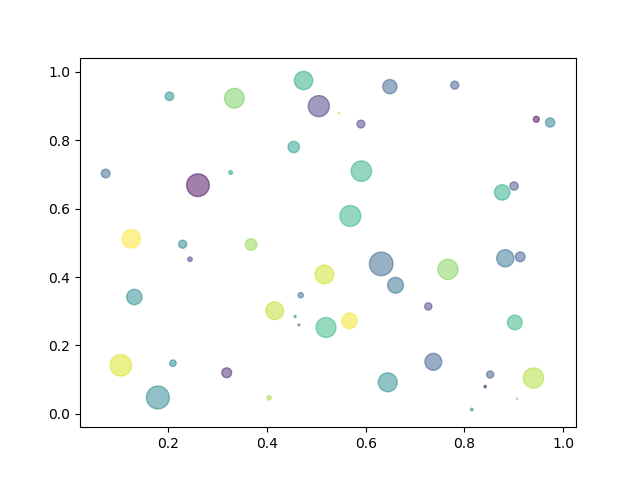1. fig = plt.figure()
5. ax1.plot(np.random.randn(50).cumsum(), 'k--')
6. ax2.hist(np.random.randn(100), bins=20, color='k')
7. ax3.scatter(np.arange(30), np.arange(30) + 3 * np.random.randn(30))
8. fig.show()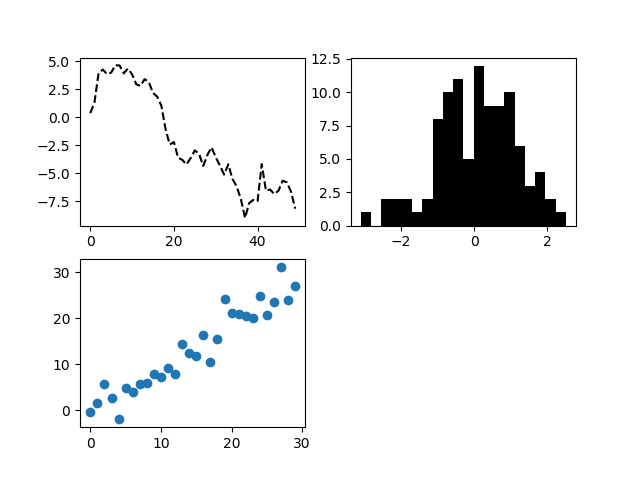## 子图的绘制

1. fig, axs = plt.subplots(2, 5, figsize=(15, 6), facecolor='w', edgecolor='k')
3. axs = axs.ravel()
4. for i in range(10):
5.     axs[i].contourf(np.random.rand(10,10),5,cmap=plt.cm.Oranges)
6.     axs[i].set_title(str(250+i))

1. axs[i].axis("off")

### 子图共享坐标轴

1. fig, (ax1, ax2) = plt.subplots(nrows=2, sharex=True)

1. fig=plt.figure()
2. ax1 = plt.subplot(211)
3. ax2 = plt.subplot(212, sharex = ax1)

1. import matplotlib.pyplot as plt
2. import numpy as np
3. t = np.arange(0.01, 5.0, 0.01)
4. s1 = np.sin(2 * np.pi * t)
5. s2 = np.exp(-t)
6. s3 = np.sin(4 * np.pi * t)
7. ax1 = plt.subplot(311)
8. plt.plot(t, s1)
9. plt.setp(ax1.get_xticklabels(), fontsize=6)
10. # share x only
11. ax2 = plt.subplot(312, sharex=ax1)
12. plt.plot(t, s2)
13. # make these tick labels invisible
14. plt.setp(ax2.get_xticklabels(), visible=False)
15. # share x and y
16. ax3 = plt.subplot(313, sharex=ax1, sharey=ax1)
17. plt.plot(t, s3)
18. plt.xlim(0.01, 5.0)
19. plt.show()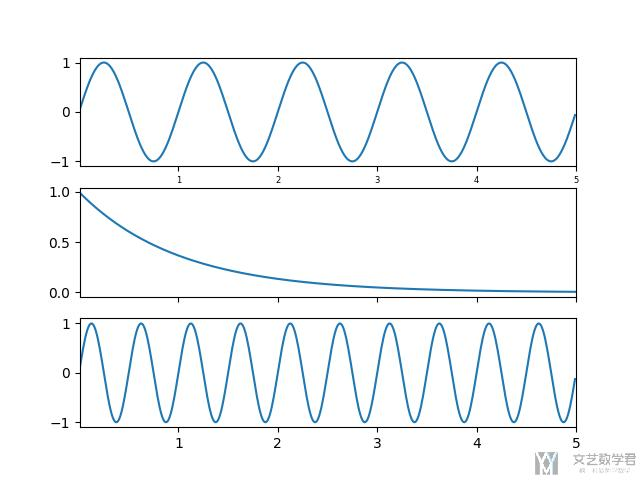## 科学绘图

1. # for lastest release (from PyPI)
2. pip install SciencePlots

1. plt.style.use(['science','ieee','no-latex'])

1. with plt.style.context(['science', 'ieee']):
2.     plt.figure()
3.     plt.plot(x, y)
4.     plt.show()

SciencePlots 默认使用 serif 字体，如果想要更换字体，可以使用下面的方式：

1. import matplotlib.pyplot as plt
2. plt.style.use('science')
3. plt.rcParams.update({
4.     "font.family": "serif",   # specify font family here
5.     "font.serif": ["Times"],  # specify font here
6.     "font.size":11})          # specify font size here

## 常用属性设置

matplotlib绘制的图形可以设置各种属性，比如设置坐标系的刻度，标题，标签等属性。 matplotplib绘制图形时，基于X, Y轴坐标系绘图。我们可以设置X, Y坐标的各种属性，比如刻度，范围，标签等属性。

### matplotlib 保存图像

1. plt.savefig("filename.png")

### matplotlib 刷新图像

1. for camera_id, camera_flow in camera_interval_flow.items():
2.     plt.cla() # 清空图片
3.     ax.plot(camera_flow) # 绘制曲线
4.     plt.savefig("{}.png".format(camera_id)) # 图像保存

### matplotlib显示中文

1. from pylab import * # 设置显示中文
2. mpl.rcParams['font.sans-serif'] = ['SimHei']
3. plt.rcParams['axes.unicode_minus']=False #用来正常显示负号

1. import matplotlib
2. a=sorted([f.name for f in matplotlib.font_manager.fontManager.ttflist])
3. for i in a:
4.     print(i)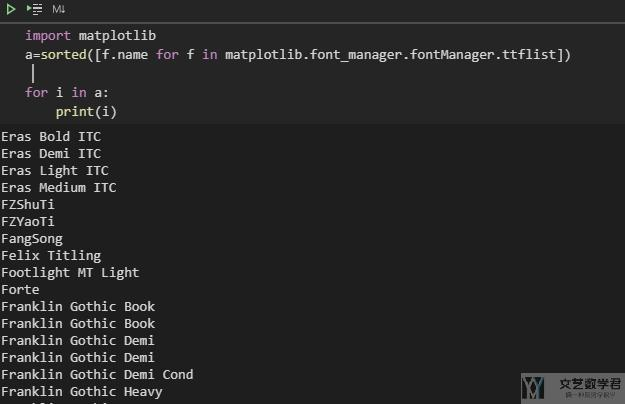### 可以显示 Latex 的公式

1. import matplotlib.pyplot as plt
2. plt.rcParams.update({
3.     "text.usetex": True,
4.     "font.family": "serif",
5.     "font.serif": ["Palatino"],
6. })

1. fig = plt.figure(figsize=(7, 5))
2. ax = fig.add_subplot(1, 1, 1)
3. menMeans = (1, 2, 3, 4, 5, 6, 7)
4. menStd = [i/5 for i in [7, 6, 5, 4, 3, 2, 1]]
5. ind = np.arange(N)    # the x locations for the groups
6. width = 0.35       # the width of the bars: can also be len(x) sequence
7. ax.bar(ind, menMeans, width, yerr=menStd)
8. plt.xticks(ind, ('$\\alpha=0.001$', '$\\alpha=0.01$', '$\\alpha=0.1$', '$\\alpha=1$', '$\\alpha=5$', '$\\alpha=10$', '$\\alpha=100$'))
9. ax.xaxis.set_tick_params(labelsize=12)
10. plt.ylim(0, 10) # 设置 y 轴的范围
11. plt.yticks(np.arange(0, 10, 0.5)) # 设置 y 轴的显示
12. ax.yaxis.set_tick_params(labelsize=12)
13. plt.show()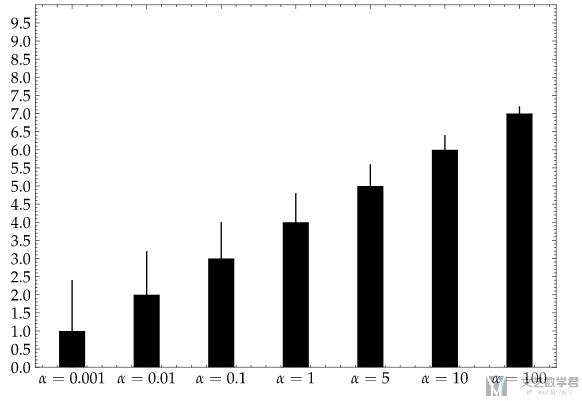### 数字与颜色的转换-map number to color

1. import matplotlib.cm as cm
2. print(cm.hot(0.3))

1. cmap = plt.get_cmap('rainbow', 12)

### 设置 x 和 y 轴的范围

1. axes = plt.gca()
2. axes.set_xlim([xmin,xmax])
3. axes.set_ylim([ymin,ymax])

### 设置标题,坐标刻度

1. fig = plt.figure()
3. # 设置标题
4. ax.set_title('Test Title')
5. major_ticks = np.arange(0, 101, 20)
6. minor_ticks = np.arange(0, 101, 5)
7. # 设置刻度
8. ax.set_xticks(major_ticks)
9. ax.set_xticks(minor_ticks, minor=True)   # 这个是短线
10. ax.set_yticks(major_ticks)
11. ax.set_yticks(minor_ticks, minor=True)
12. # 设置 X, Y 轴 标签
13. ax.set_xlabel("X axis")
14. ax.set_ylabel("Y axis")
15. # 设置网格
16. ax.grid(which='minor', alpha=0.2)
17. ax.grid(which='major', alpha=0.5)
18. # 添加文字
19. ax.text(42.5, 50, "Hello World")
20. fig.show()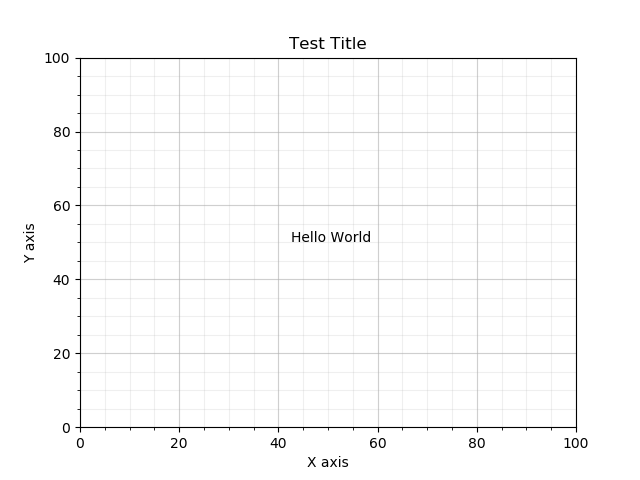1. ax.set_xticks([])
2. ax.set_yticks([])

1. ax1.grid(axis='y', which='major', alpha=0.4, linestyle='--')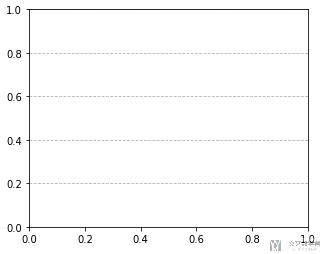### 设置标题位置

1. fig, ax = plt.subplots(figsize=(6, 3), subplot_kw=dict(aspect="equal"))
2. ax.set_title("Title Position")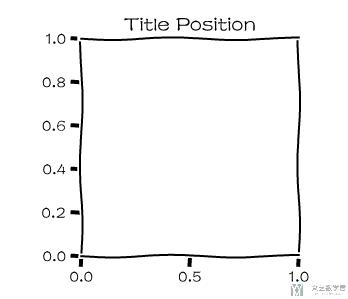1. fig, ax = plt.subplots(figsize=(6, 3), subplot_kw=dict(aspect="equal"))
2. ax.set_title("Title Position", y=1.5)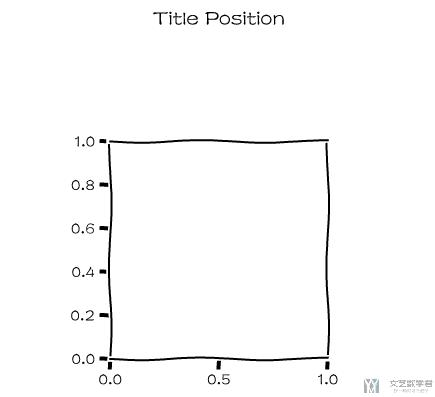### 加入文字

1. text(x, y, s, bbox=dict(facecolor='red', alpha=0.5))

1. ax.text(np.mean(v_x[ix][:,0]), np.mean(v_x[ix][:,1]), key, fontsize=18, bbox=dict(facecolor='white', alpha=0.5))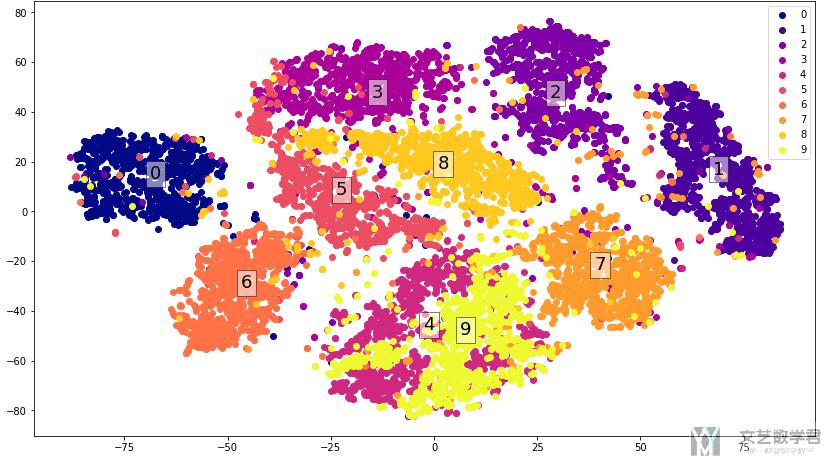### 设置坐标轴范围

1. fig = plt.figure(figsize=(7, 5))
2. ax = fig.add_subplot(1, 1, 1)
3. plt.xlim(0, 10) # 设置 x 轴的范围
4. plt.xticks(np.arange(0, 10, 2)) # 设置 x 轴的显示
5. plt.ylim(0, 10) # 设置 y 轴的范围
6. plt.yticks(np.arange(0, 10, 0.5)) # 设置 y 轴的显示
7. ax.yaxis.set_tick_params(labelsize=12)
8. plt.show()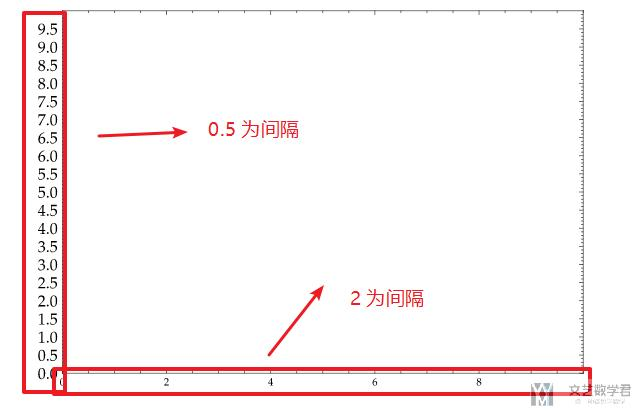### 设置坐标轴刻度与坐标轴名称

1. from pylab import * # 设置显示中文
2. mpl.rcParams['font.sans-serif'] = ['SimHei']
3. plt.rcParams['axes.unicode_minus']=False #用来正常显示负号
4. x = np.linspace(0, 1, 100)
5. fig = plt.figure(figsize=(12,8))
7. ax.set_title(u'设置曲线颜色与图例', fontsize=17)
8. # 绘制图形
9. ax.plot(x, x ** 2, 'b.', label=r'$y = x^{2}$')
10. # 设置坐标轴的标签
11. ax.yaxis.set_tick_params(labelsize=15) # 设置y轴的字体的大小
12. ax.set_xticks(np.linspace(0, 1, 11)) # 设置xticks出现的位置
13. xticks = [i if i in [0, 0.5, 0.8, 1.0] else '' for i in np.linspace(0, 1, 11)] # 只显示特定数字
14. ax.set_xticklabels(xticks, rotation=-45, fontsize=17) # 设置xticks的值
15. # 设置坐标轴名称
16. ax.set_ylabel("这是Y轴", fontsize='xx-large')
17. ax.set_xlabel('这是X轴',  fontsize='xx-large')
18. # 自动生成图例
19. ax.legend(fontsize=17)
20. # 设置图像x,y轴的范围，都是从0-1
21. ax.axis([0, 1, 0, 1])
22. fig.show()

• 设置坐标轴刻度的间隔；
• 设置坐标轴刻度的名称，调整大小和倾斜角度；
• 设置 x 轴，y 轴的名称；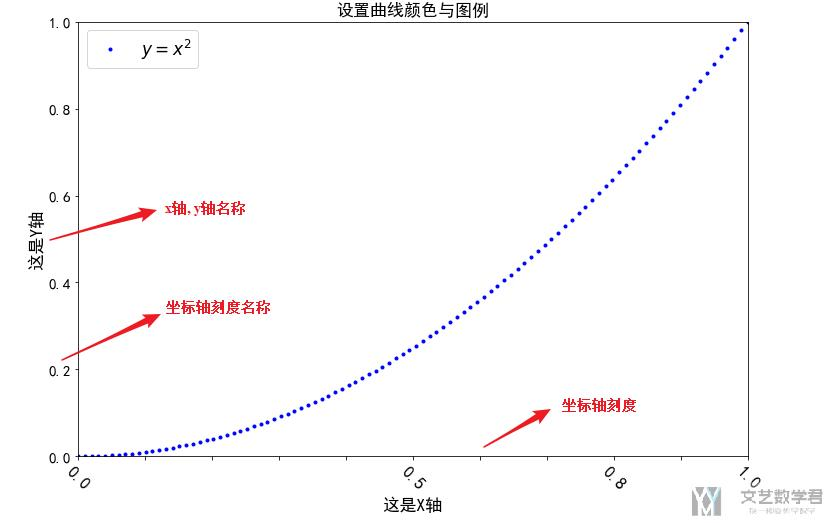### 设置坐标轴为log scale

1. dt = 0.01
2. x = np.arange(-50.0, 50.0, dt)
3. y = np.arange(0, 100.0, dt)
4. fig = plt.figure()
6. ax.plot(x, y)
7. # 设置y scale
8. plt.yscale('log')
9. plt.ylabel('logy') # 设置y轴的label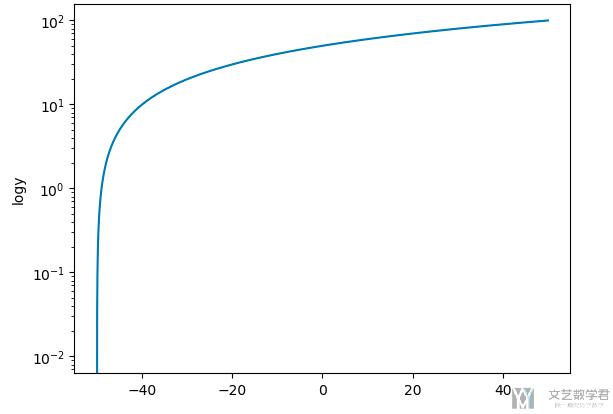### 设置曲线颜色，图例

1. # 设置显示中文
2. from pylab import *
3. mpl.rcParams['font.sans-serif'] = ['SimHei']
4. plt.rcParams['axes.unicode_minus']=False #用来正常显示负号
5. x = np.linspace(0, 1,100)
6. fig = plt.figure()
8. ax.set_title(u'设置曲线颜色与图例')
9. # 四个参数分别表示x,y轴数据,'b--'表示颜色是蓝色样式是虚线,最后一个表示标签，支持LaTex
10. ax.plot(x, x ** (1/8), 'b--', label=r'$y = x^{1/8}$')
11. ax.plot(x, x ** 8, 'r--', label=r'$y = x^{8}$')
12. ax.plot(x, x ** (1/2), 'r.', label=r'$y = x^{1/2}$')
13. ax.plot(x, x ** 2, 'b.', label=r'$y = x^{2}$')
14. ax.plot(x, x, 'g-', label=r'$y = x$')
15. # 自动生成图例
16. ax.legend()
17. # 设置图像x,y轴的范围，都是从0-1
18. ax.axis([0, 1, 0, 1])
19. fig.show()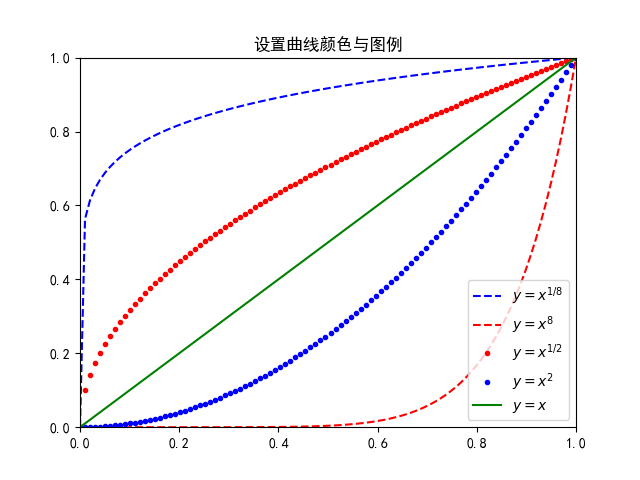『线的样式』linestyle=

• '-' 默认实线
• '--' 虚线
• '-.' 间断线
• ':' 点状线

• 'b' blue
• 'g' green
• 'r' red
• 'c' cyan
• 'm' magenta
• 'y' yellow
• 'k' black（黑色）
• 'w' white

### 图例与 pandas 结合使用

1. plt.legend(legend.values,loc=4)

### 关于图例位置自定义

1. from pylab import * # 设置显示中文
2. mpl.rcParams['font.sans-serif'] = ['SimHei']
3. plt.rcParams['axes.unicode_minus']=False #用来正常显示负号
4. x = np.linspace(0, 1,100)
5. fig = plt.figure(figsize=(12,8))
7. ax.set_title(u'设置曲线颜色与图例')
8. # 四个参数分别表示x,y轴数据,'b--'表示颜色是蓝色样式是虚线,最后一个表示标签，支持LaTex
9. ax.plot(x, x ** 8, 'r--', label=r'$y = x^{8}$')
10. ax.plot(x, x ** 2, 'b.', label=r'$y = x^{2}$')
11. # 手动设置图例
12. ax.text(x[-10], 0.9*(x ** 8) [-10], r'$y = x^{8}$', fontsize=17)
13. ax.text(x[-26], 1.2*(x ** 2) [-26], r'$y = x^{2}$', fontsize=17)
14. # 自动生成图例
15. ax.legend()
16. # 设置图像x,y轴的范围，都是从0-1
17. ax.axis([0, 1, 0, 1])
18. fig.show()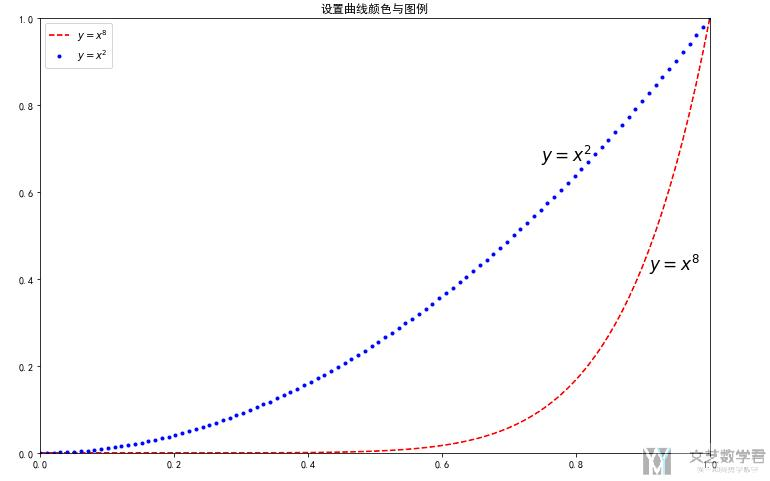## 绘制图像的样式

### 绘制xkcd样式图片

1. plt.xkcd()
2. fig = plt.figure()
3. ax = fig.add_subplot(1, 1, 1)
4. np.random.seed(7)
5. x = np.linspace(1, 10, 50)
6. y = 2*x + 0.5*x**2 + 0.3*x**4 - 0.025*x**5 + 5*np.random.rand(50)
7. ax.scatter(x, y)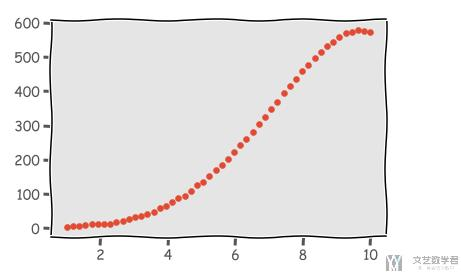1. with plt.xkcd():
2.     # This figure will be in XKCD-style
3.     fig1 = plt.figure()
4.     # ...
5. # This figure will be in regular style
6. fig2 = plt.figure()

1. plt.xkcd()
2. plt.rcParams['font.family'] = ['FZShuTi']

1. plt.xkcd()
2. plt.rcParams['font.family'] = ['FZShuTi'] # 这个需要在设置样式之后再设置
3. fig = plt.figure()
4. ax = fig.add_subplot(1, 1, 1)
5. ax.set_title(u'是否考虑自己未来的规划', fontsize=25)
6. x = np.arange(3)*1.2
7. y = [137, 140, 176] # 有
8. z = [33, 45, 38] # 没有
9. sum = [i+j for i,j in zip(y,z)] # 计算每个年纪所占的比例
10. y =  [i/j for i,j in zip(y,sum)]
11. z = [i/j for i,j in zip(z,sum)]
12. w=0.3
13. # 绘制多个bar在同一个图中, 这里需要控制width
14. plt.bar(x-w/2, y, width=w, align='center', label='有')
15. plt.bar(x+w/2, z, width=w, align='center', label='没有')
16. ax.set_xticks(x) # 设置xticks出现的位置
17. ax.set_xticklabels(['高一', '高二', '高三'], rotation='30', fontsize='xx-large')
18. ax.plot(x, y, c='green') # 画出曲线
19. ax.legend(bbox_to_anchor=(1, 0.95)) # 绘制图例
20. plt.show()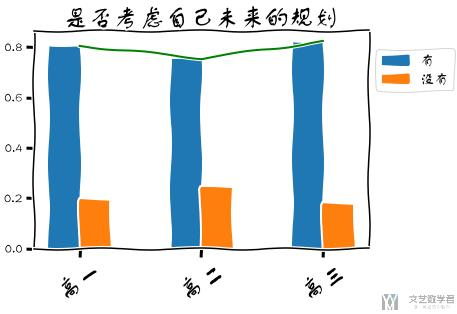### 图像的样式概览

1. # 设置画图的style
2. matplotlib.style.use('default')

matplotlib风格类型: Style sheets reference

### 绘制其他样式

1. # 生成随机数据
2. np.random.seed(7)
3. x = np.linspace(1, 12, 10)
4. y = 2*x + 0.7*x**2 - 20*np.sin(x) - 20*np.cos(x) + 5*np.random.rand(10)
5. plt.style.use("ggplot") # 使用美观的样式
6. plt.scatter(x, y)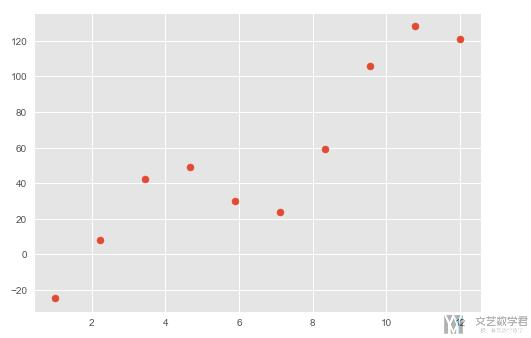### 设置图像颜色风格

1. plt.set_cmap('RdBu')

1. x = np.arange(3)*1.2
2. y = [4, 9, 2]
3. z = [1, 2, 3]
4. k = [11, 12, 13]
5. ax = plt.subplot(111)
6. plt.set_cmap('RdBu')
7. w=0.3
8. # 绘制多个bar在同一个图中, 这里需要控制width
9. plt.bar(x-w, y, width=w, align='center')
10. plt.bar(x, z, width=w, align='center')
11. plt.bar(x+w, k, width=w, align='center')
12. ax.set_xticks(x) # 设置xticks出现的位置
13. ax.set_xticklabels(['1','2','3'], rotation='vertical', fontsize = 'xx-large')
14. ax.autoscale(tight=True)
15. plt.show()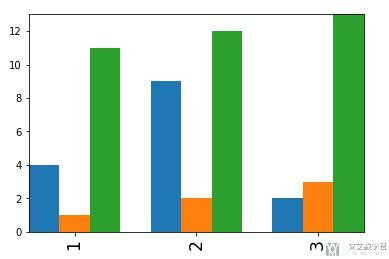### 设置图像颜色

• #e0109c
• #1b71f1
• #9eed3b
1. x = np.arange(3)*1.2
2. y = [4, 9, 2]
3. z = [1, 2, 3]
4. k = [11, 12, 13]
5. ax = plt.subplot(111)
6. w=0.3
7. # 绘制多个bar在同一个图中, 这里需要控制width
8. plt.bar(x-w, y, width=w, color='#e0109c', align='center')
9. plt.bar(x, z, width=w, color='#1b71f1', align='center')
10. plt.bar(x+w, k, width=w, color='#9eed3b', align='center')
11. ax.set_xticks(x) # 设置xticks出现的位置
12. ax.set_xticklabels(['1','2','3'], rotation='vertical', fontsize = 'xx-large')
13. ax.autoscale(tight=True)
14. plt.show()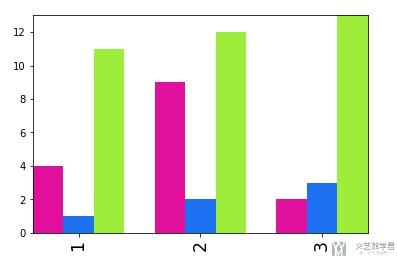## 常用图像

### 水平线

1. import seaborn as sns
2. import matplotlib.pyplot as plt
3. import numpy as np
4. import pandas as pd
5. x = np.array([2, 2, 4, 4])
6. y = np.array([5, 10, 10, 15])
7. isBool = np.array([TrueFalseTrueFalse])
8. data = pd.DataFrame(
9.     np.column_stack((x, y, isBool)),
10.     columns=["x", "y", "someBoolean"]
11. )
12. ax = sns.lineplot(x="x", y="y", hue="someBoolean", data=data)
13. ax.axhline(y=7, c='red', linestyle='dashed', label="h7")
14. ax.axhline(y=15, c='blue', linestyle='dashed', label="h15")
15. plt.legend()
16. plt.show()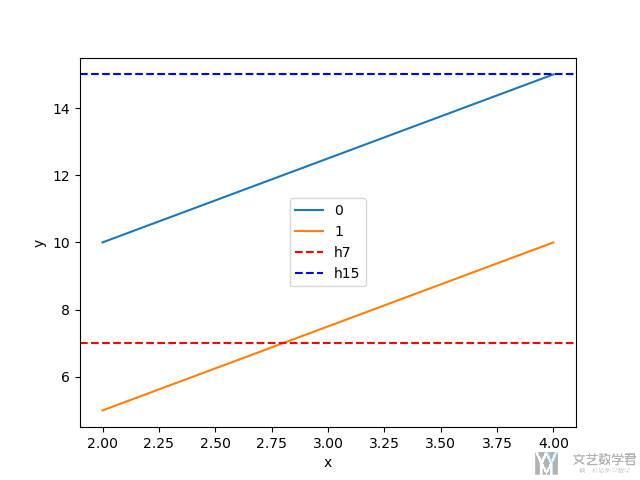### 线形图（折线图）

1. fig = plt.figure()
3. x = np.arange(10)
4. y = x**2
5. ax.plot(x,y)
6. fig.show()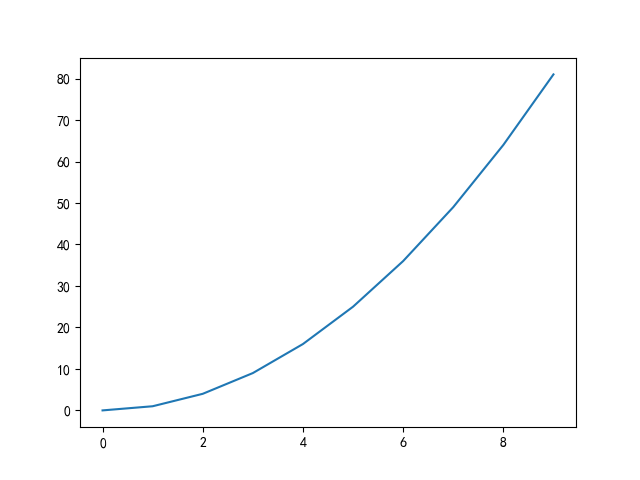下面我们看一个稍微复杂的折线图，完整的代码如下：
1. plt.style.use(['science','ieee','no-latex']) # 设置格式
2. fig, ax = plt.subplots(figsize=(6, 4), nrows=1,ncols=1)
3. # 定义数据
4. x_indexs = [0, 1, 2, 3, 4, 5]
5. heights = [0.98, 0.93, 0.83, 0.72, 0.68, 0.66]
6. # 绘图
7. ax.plot(x_indexs, heights, '--', linewidth=2, marker='o', markersize=4) # 绘制折线图
8. # 绘制竖线
9. for x_index, height in zip(x_indexs,heights):
10.     ax.plot([x_index, x_index], [0, height], 'k--', linewidth=0.5)
11. # 设置图的样式
12. ax.set(ylim=[0.5, 1]) # 设置 y 轴范围
13. ax.set_xticks(x_indexs) # 设置xticks出现的位置
14. ax.set_xticklabels(['$\gamma$=0.01','$\gamma$=0.1','$\gamma$=0.2', '$\gamma$=0.5', '$\gamma$=0.9', '$\gamma$=0.99'])
15. ax.set_ylabel('F-measure')

### 绘制误差区间

1. import numpy as np
2. import matplotlib.pyplot as plt
3. import seaborn as sns
4. if __name__ == '__main__':
5.     data =  np.random.random((3, 5, 20))
6.     data +=  5
7.     data +=  10
8.     # Reward 与时间的关系
9.     clrs = sns.color_palette("husl", 5)
10.     fig = plt.figure(figsize=plt.figaspect(0.6))
12.     labels = ['A', 'B', 'C']
13.     for i, label in enumerate(labels):
14.         steps = list(range(20))
15.         mean = np.mean(data[i], 0) # 计算均值
16.         std = np.std(data[i], 0) # 计算方差
17.         ax.plot(steps, mean, label=label, c=clrs[i])
18.         ax.fill_between(steps, mean-std, mean+std ,alpha=0.3, facecolor=clrs[i])
19.     plt.legend()
20.     plt.show()

### 绘制箭头

1. fig = plt.figure(figsize=plt.figaspect(1))
3. ax1.arrow(x=0, y=0, dx=0.5, dy=0.5, width=0.01)
4. plt.show()

### 柱状图

#### 纵向的柱状图

1. fig = plt.figure(figsize=(7,5))
3. bar_data = [5, 20, 15, 25, 10]
4. bar_labels = ['Tom', 'Dick', 'Harry', 'Slim', 'Jim']
5. plt.bar(range(len(bar_data)), bar_data, tick_label=bar_labels)
6. plt.show()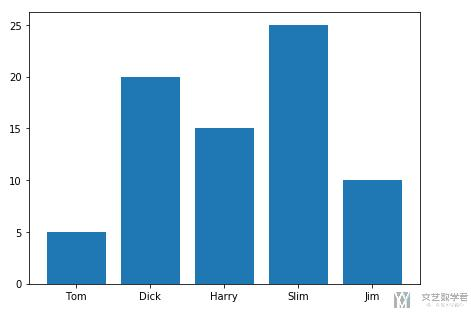#### 堆叠柱状图

1. data = pd.DataFrame({'A': [1,3,2,4], 'B': [4,2,3,1], 'index': ['test1', 'test2', 'test3', 'test4']})

1. plt.rcParams['figure.figsize'] = (10, 6)
2. plt.bar(x='index', height='A', data=data, width=1.0, label='下')
3. plt.bar(x='index', height='B', bottom='A', data=data, width=1, label='上')
4. ax = plt.gca()
5. ax.legend(prop=myfont, bbox_to_anchor=(1.0, 0.9))

#### 绘制多条柱状图

1. x = np.arange(3)*1.2
2. y = [4, 9, 2]
3. z = [1, 2, 3]
4. k = [11, 12, 13]
5. ax = plt.subplot(111)
6. w=0.3
7. # 绘制多个bar在同一个图中, 这里需要控制width
8. plt.bar(x-w, y, width=w, color='#e0109c', align='center')
9. plt.bar(x, z, width=w, color='#1b71f1', align='center')
10. plt.bar(x+w, k, width=w, color='#9eed3b', align='center')
11. ax.set_xticks(x) # 设置xticks出现的位置
12. ax.set_xticklabels(['1','2','3'], rotation='vertical', fontsize = 'xx-large')
13. ax.autoscale(tight=True)
14. plt.show()

#### 包含标准差的柱状图

1. fig = plt.figure(figsize=(7, 5))
2. ax = fig.add_subplot(1, 1, 1)
3. menMeans = (1, 2, 3, 4, 5, 6, 7)
4. menStd = [i/5 for i in [7, 6, 5, 4, 3, 2, 1]]
5. ind = np.arange(N)    # the x locations for the groups
6. width = 0.35       # the width of the bars: can also be len(x) sequence
7. ax.bar(ind, menMeans, width, yerr=menStd)
8. plt.xticks(ind, ('$\\alpha=0.001$', '$\\alpha=0.01$', '$\\alpha=0.1$', '$\\alpha=1$', '$\\alpha=5$', '$\\alpha=10$', '$\\alpha=100$'))
9. ax.xaxis.set_tick_params(labelsize=12)
10. plt.ylim(0, 10) # 设置 y 轴的范围
11. plt.yticks(np.arange(0, 10, 0.5)) # 设置 y 轴的显示
12. ax.yaxis.set_tick_params(labelsize=12)
13. plt.show()

1. ax.bar(ind, menMeans, width, color='k', yerr=menStd, error_kw=dict(elinewidth=2, ecolor='#7f8c8d', capsize=5))

1. ax.barh(x_ticks, data_mean, xerr=data_std, error_kw=dict(elinewidth=2, ecolor='#7f8c8d', capsize=3))

#### 使用柱状图和折线图显示累计占比

1. binRange = [0, 784, 1024, 4096, 100000]
2. sample_num, _ = np.histogram(pcaps_size_list, bins=binRange)
3. print(sample_num)
4. """
5. [46054  1413  3437  3691]
6. """

1. sample_num_percentage = np.cumsum(sample_num, dtype=float)/sum(sample_num)
2. print(sample_num_percentage)
3. """
4. [0.8435571  0.86943859 0.93239308 1.        ]
5. """

1. # 绘制直方图和折线图
2. fig = plt.figure()
4. fig_index = ['0-784', '784-1024', '1024-4096', '>4096']
5. # 绘制柱状图
6. ax.bar(fig_index, sample_num)
7. ax.set_ylim([0, 70000]) # 设置 y 轴范围
8. ax.set_xlabel('Pcap Size (Byte)') # 设置 x 轴的 label
9. ax.set_ylabel('Pcap Number') # 设置 y 轴的 label
10. # 绘制折线图
11. ax_twin = ax.twinx() # 次坐标
12. ax_twin.plot(fig_index, sample_num_percentage, marker='o', markersize=3)
13. ax_twin.set_ylim([0, 1.1])
14. # 添加文字
15. for tmp_index, tmp_percentage in zip(fig_index, sample_num_percentage):
16.     ax_twin.text(tmp_index, 1.03* tmp_percentage, "{:<6.2f}".format(tmp_percentage))

### 饼图的绘制

#### 基础饼图的绘制

1. # Pie chart, where the slices will be ordered and plotted counter-clockwise:
2. labels = 'Frogs', 'Hogs', 'Dogs', 'Logs'
3. sizes = [15, 30, 45, 10]
4. explode = (0, 0.1, 0, 0)  # only "explode" the 2nd slice (i.e. 'Hogs')
5. fig1, ax1 = plt.subplots()
6. ax1.pie(sizes, explode=explode, labels=labels, autopct='%1.1f%%',
8. ax1.axis('equal')  # Equal aspect ratio ensures that pie is drawn as a circle.
9. plt.show()

#### 对饼图进行 Label

1. fig, ax = plt.subplots(figsize=(7, 5), subplot_kw=dict(aspect="equal"))
2. y1 = [135, 148, 166]
3. y3 = [73, 95, 93]
4. y4 = [85, 99, 82]
5. y5 = [114, 121, 122]
6. y6 = [105, 107, 100]
7. data = [np.sum(i) for i in [y1, y2, y3, y4, y5, y6]]
8. title = ["开设职业生涯规划课程",
9.           "举办职业规划讲座",
10.           "在学校开通职业规划评价系统",
11.           "在学校设立专门的职业指导中心",
12.           "创建丰富多彩的职业体验课程",
13.           "加强教师和家长的指导"]
14. recipe = ['{} {:<3.2f} %'.format(i,j/np.sum(data)*100) for i,j in zip(title, data)] # 设置标题
15. # 下面的不需要修改
16. wedges, texts = ax.pie(data, wedgeprops=dict(width=0.4), startangle=-40)
17. bbox_props = dict(boxstyle="square,pad=0.3", fc="w", ec="k", lw=0.72)
18. kw = dict(arrowprops=dict(arrowstyle="-"),
19.           bbox=bbox_props, zorder=0, va="center")
20. for i, p in enumerate(wedges):
21.     ang = (p.theta2 - p.theta1)/2. + p.theta1
24.     horizontalalignment = {-1: "right", 1: "left"}[int(np.sign(x))]
25.     connectionstyle = "angle,angleA=0,angleB={}".format(ang)
26.     kw["arrowprops"].update({"connectionstyle": connectionstyle})
27.     ax.annotate(recipe[i], xy=(x, y), xytext=(1.35*np.sign(x), 1.4*y),
28.                 horizontalalignment=horizontalalignment, **kw)
29. ax.set_title(u"您认为学校开展职业生涯规划教育的措施有哪些", fontsize=25, y=1.2)
30. plt.show()

• 关于 label 的位置，我们可以通过 xytext 来控制，及上面的 1.35*np.sign(x), 和 1.4*y, 可以控制前面的系数, 1.35 和 1.4，来使我们的 label 的位置不一样；
• 控制 label 的字体大小，可以直接在 axannotate 部分增加参数 fontsize 即可。如下面的例子：
1. for x, y, label, size in zip(X, Y, labels, sizes):
2.     ax.annotate(label, (x, y), fontsize=size)

### 直方图

1. fig = plt.figure()
3. data = np.random.normal(0,20,1000) # 在0-20之间产生1000个随机数
4. bins = np.arange(-100,100,5) # 产生区间刻度
5. ax.hist(data,bins=bins)
6. fig.show()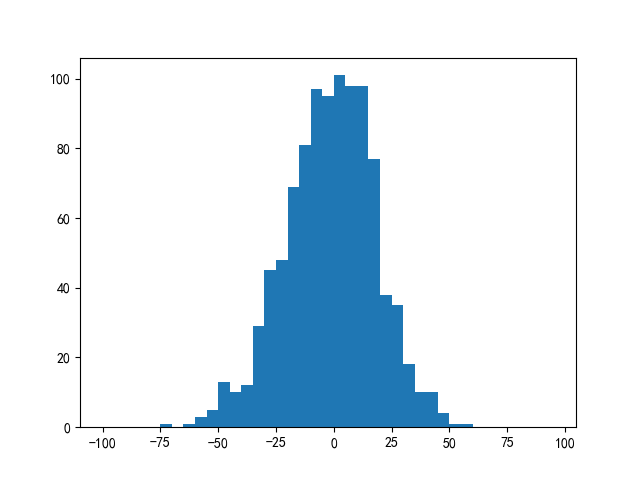我们也可以将多个直方图绘制在一起，同时可以加上直方图的密度曲线，我们看下面的一个例子：
1. import random
2. from scipy import stats
3. # 产生测试数据
4. x = [random.gauss(3,1) for _ in range(400)]
5. y = [random.gauss(4,2) for _ in range(400)]
6. # 拟合密度曲线
7. kde1 = stats.gaussian_kde(x)
8. kde2 = stats.gaussian_kde(y)
9. bins = np.linspace(-10, 10, 100)
10. # 绘制直方图
11. plt.hist(x, bins, alpha=0.5, label='x', normed=True, color='#2980b9')
12. plt.hist(y, bins, alpha=0.5, label='y', normed=True, color='#f39c12')
13. # 绘制曲线
14. plt.plot(bins, kde1(bins), lw=4, color='#2980b9')
15. plt.plot(bins, kde2(bins), lw=4, color='#f39c12')
16. plt.legend(loc='upper right')
17. plt.show()

### 散点图

1. fig = plt.figure()
3. x = np.arange(1,100,1)
4. y = x**2+100*x*np.random.random(len(x))
5. ax.scatter(x,y)
6. fig.show()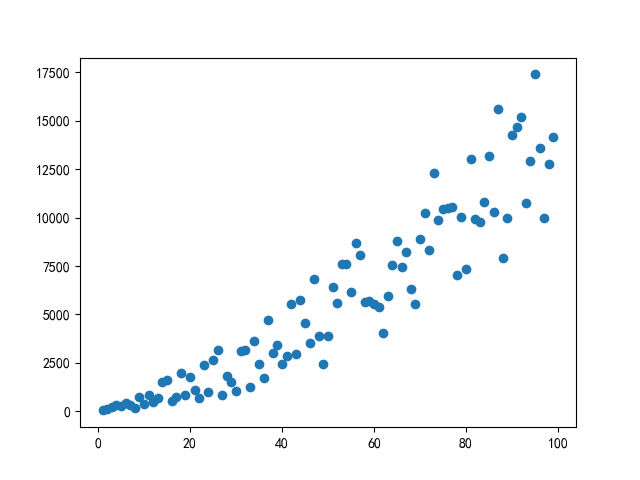#### 散点图的美化

1. data = []
2. years = [str(i) for i in list(range(1990, 2020, 1))] # 所有的年份
3. ages = [str(i) for i in list(range(0, 81, 5))] # 所有的年龄段
4. populations = [10000*i for i in list(range(0, 81, 5))] # 每个年龄段的人口
5. for year_index, year in enumerate(years):
6.     for age_index, age in enumerate(ages):
7.         data.append([year, age, (1+0.1*year_index)*populations[age_index]])
8. plot_data = pd.DataFrame(data, columns=['year', 'age', 'population'])

1. plt.rcParams['figure.figsize'] = (18, 8)
2. plt.scatter(
3.     x=plot_data['year'],
4.     y=plot_data['age'],
5.     c=plot_data['population'],
6.     s=plot_data['population']*0.0005,
7.     ec='tab:gray',
8.     cmap=plt.cm.RdBu_r,
9.     alpha=0.8)
10. ax = plt.gca()
11. # 坐标轴控制
12. ax.set_xticks([years[i] for i in range(0, len(years), int(len(years)/5))])
13. ax.set_yticks([ages[i] for i in range(0, len(ages), int(len(ages)/5))])
14. # 不显示左侧和底部纵坐标轴
15. ax.spines['left'].set_visible(False)
16. ax.spines['bottom'].set_visible(False)
17. # 显示 y 轴网格线
18. ax.grid(b=False, axis='y', lw=1)
19. plt.ylabel('Mean Age')

#### 高阶散点图-指定不同类别使用不同颜色

1. cmap = plt.get_cmap('rainbow', 12) # 数字与颜色的转换
2. scatter_x = np.array([1,2,3,4,5])
3. scatter_y = np.array([5,4,3,2,1])
4. group = np.array([1,3,2,1,3])
5. cdict = {1: '111', 2: '222', 3: '333'}
6. fig, ax = plt.subplots()
7. for key in cdict.keys():
8.     ix = np.where(group == key)
9.     ax.scatter(scatter_x[ix], scatter_y[ix], color = cmap(key), label = key, s = 100) # 注意这里color的指定
10. ax.legend()
11. plt.show()

1. markers = ["8","s","p","P","*","h","H","+","x","X","D","d"]

### 绘制多边形(区间的绘制)

1. # 绘制一个多边形的区域
2. test_x = np.array([1,2,3])
3. test_y = np.array([4,1,2])
4. fig, axes = plt.subplots(nrows=1, ncols=1,figsize=(13,7))
5. axes.fill(test_x,test_y)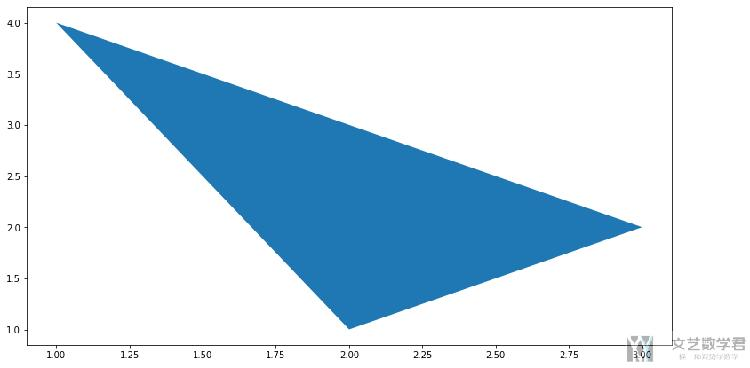我们可以使用这个方法来绘制一个区间的效果；
1. # 绘制一个多边形的区域
2. test_x = np.linspace(0.1, 9.9, 200)
3. test_y = np.sin(test_x)
4. fig, axes = plt.subplots(nrows=1, ncols=1,figsize=(13,7))
5. axes.plot(test_x,test_y,c='r')
6. axes.fill(np.concatenate([test_x,test_x[::-1]]),np.concatenate([test_y + 1, test_y - 1]))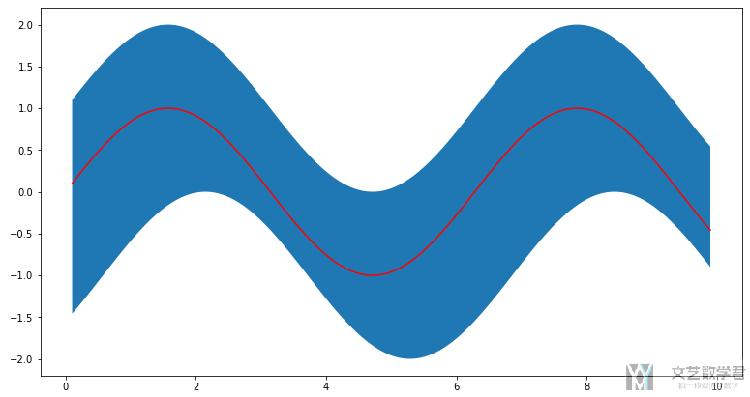### 箱线图

• 最小值；
• 第1四分位数；
• 中位数；
• 第3四分位数；
• 最大值；

1. fig = plt.figure()
3. # 产生 50 个小于 100 的随机数
4. spread = np.random.rand(50) * 100
5. # 产生 25 个值为 50 的数据
6. center = np.ones(25) * 50
7. # 异常值
8. outlier_high = np.random.rand(10) * 100 + 100
9. outlier_low = np.random.rand(10) * -100
10. data = np.concatenate((spread, center, outlier_high, outlier_low), 0)
11. ax.boxplot(data)
12. fig.show()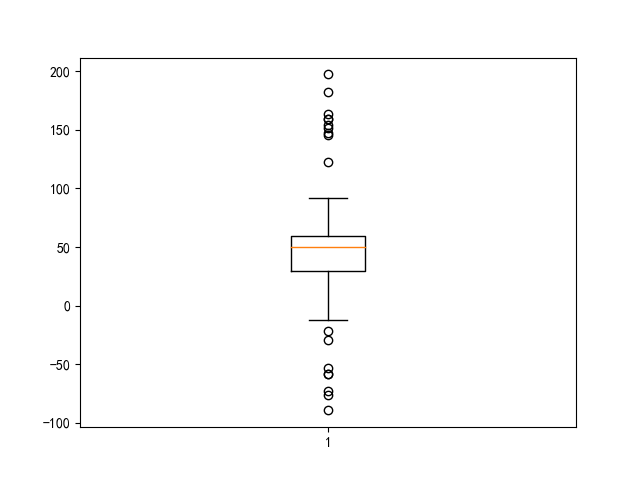1. fig1, ax1 = plt.subplots(figsize=(16,8))
2. # 产生数据
3. test1 = np.random.randint(100, 200, 1000)
4. test2 = np.random.randint(300, 400, 1000)
5. ax1.boxplot([test1, test2], labels=['test1', 'tets2'])
6. ax1.set_xticklabels(['test1', 'tets2'], rotation=-45, fontsize=18)
7. ax1.yaxis.set_tick_params(labelsize=18)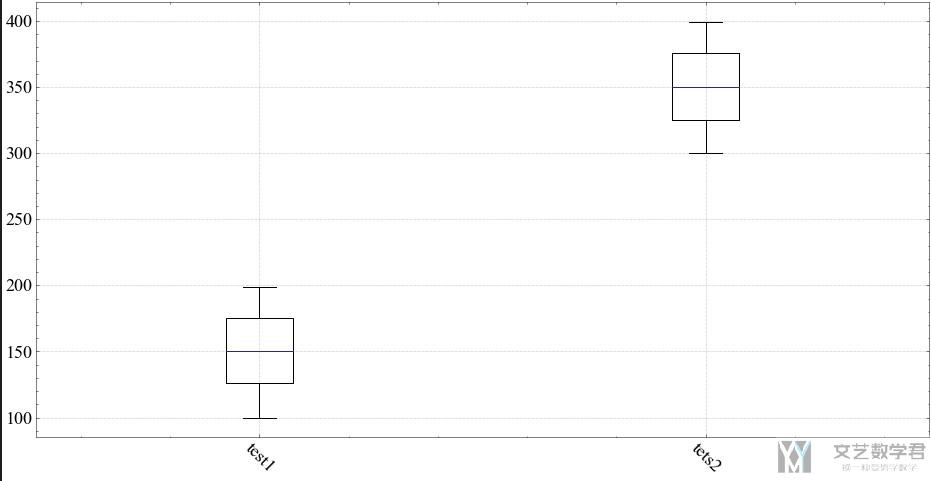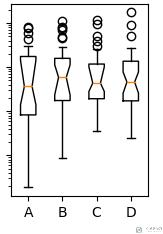## 绘制动态图

### 简单的动画制作

1. import numpy as np
2. import matplotlib.pyplot as plt
3. import matplotlib.animation as animation
4. fig = plt.figure()
6. x = np.arange(0,2*np.pi,0.01)
7. line, = plt.plot(x,np.sin(x))
8. # 更新函数
9. def update(i):
10.     line.set_ydata(np.sin(x+i/10))
11.     return line
12. animation = animation.FuncAnimation(fig,update,interval=20)
13. plt.show()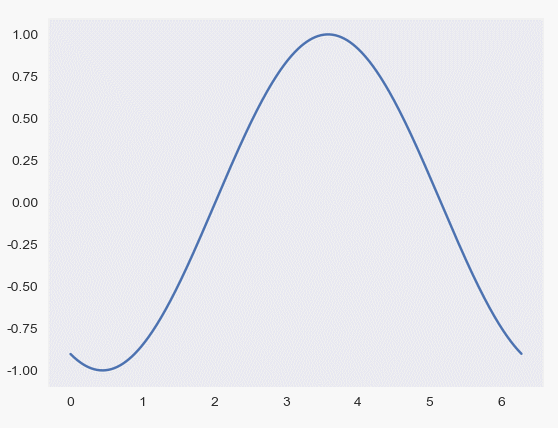### 通过更新列表更新图像

1. import matplotlib.pyplot as plt
2. import matplotlib.animation as animation
3. fig = plt.figure()
4. #creating a subplot
6. Date = [1,2,3,4,5,6,7,8,9]
7. Price = [100, 120, 320, 370, 420, 600, 550, 720, 900]
8. def animate(i):
9.     xs = Date[:i]
10.     ys = Price[:i]
11.     print(xs, ys)
12.     ax1.clear() # 清除画布
13.     ax1.plot(xs, ys)
14.     plt.xlabel('Date')
15.     plt.ylabel('Price')
16.     plt.title('Live graph with matplotlib')
17. ani = animation.FuncAnimation(fig, animate, interval=500)
18. plt.show()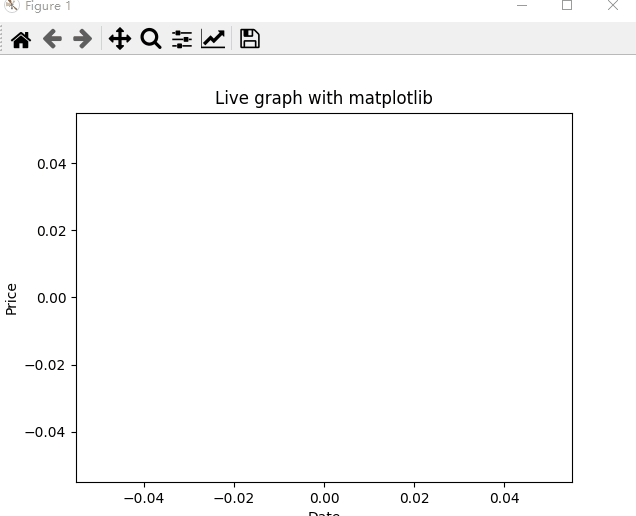## 绘制三维图像

### 绘制三维散点图

1. import numpy as np
2. # 导入2d,3d的绘图模块
3. from mpl_toolkits.mplot3d import Axes3D
4. import matplotlib.pyplot as plt
5. # x, y, z 均为 0 到 1 之间的 100 个随机数
6. x = np.random.normal(0, 1, 100)
7. y = np.random.normal(0, 1, 100)
8. z = np.random.normal(0, 1, 100)
9. fig = plt.figure()
10. ax = Axes3D(fig)
11. ax.scatter(x,y,z)
12. plt.show()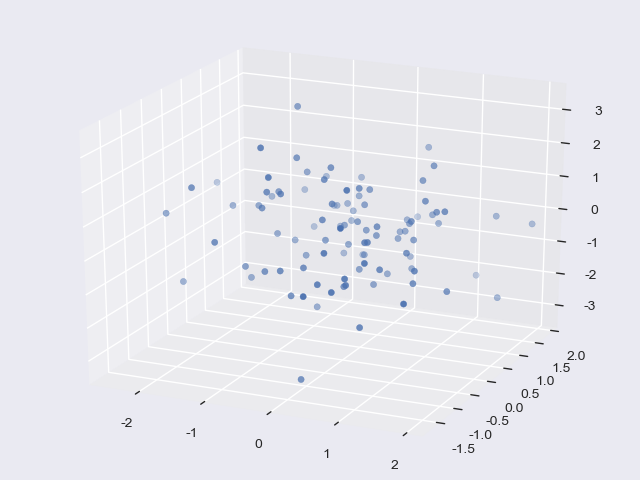### 绘制三维线形图

1. # 载入模块
2. from mpl_toolkits.mplot3d import Axes3D
3. import matplotlib.pyplot as plt
4. import numpy as np
5. # 生成数据
6. x = np.linspace(-6 * np.pi, 6 * np.pi, 1000)
7. y = np.sin(x)
8. z = np.cos(x)
9. # 创建 3D 图形对象
10. fig = plt.figure()
11. ax = Axes3D(fig)
12. # 绘制线型图
13. ax.plot(x, y, z)
14. # 显示图
15. plt.show()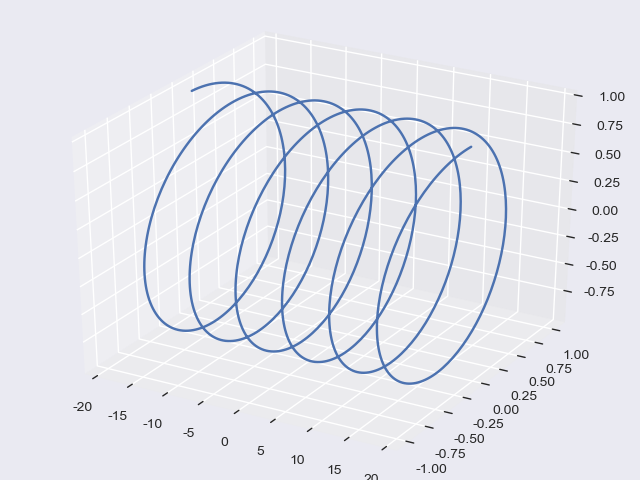### 绘制三维曲面图

1. # 载入模块
2. import numpy as np
3. import matplotlib.pyplot as plt
4. from mpl_toolkits.mplot3d import Axes3D
5. # 创建 3D 图形对象
6. fig = plt.figure()
7. ax = Axes3D(fig)
8. # 生成数据
9. X = np.arange(-2, 2, 0.1)
10. Y = np.arange(-2, 2, 0.1)
11. X, Y = np.meshgrid(X, Y) #注意这一步
12. Z = np.sqrt(X ** 2 + Y ** 2)
13. # 绘制曲面图，并使用 cmap 着色
14. ax.plot_surface(X, Y, Z, cmap=plt.cm.winter)
15. plt.show()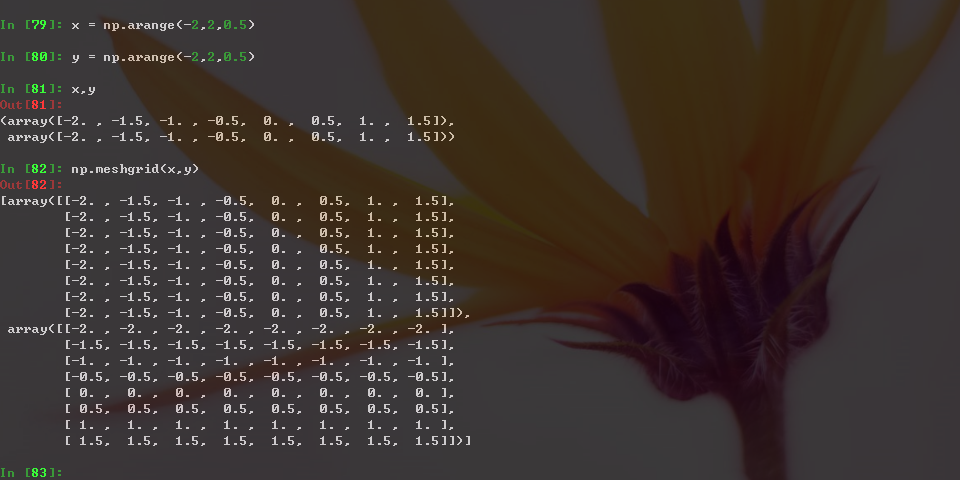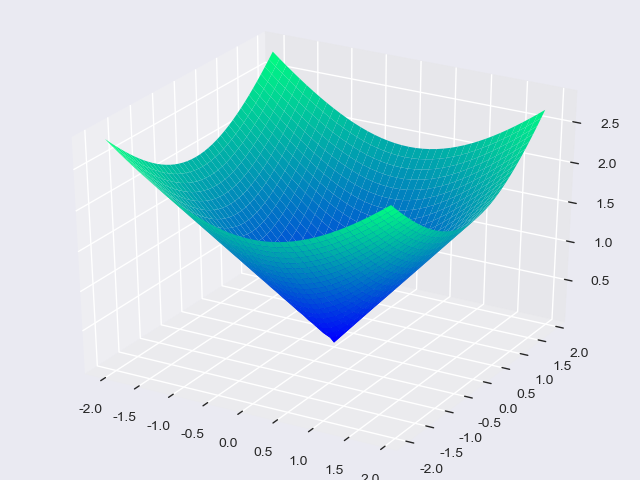### 子图的绘制

1. # -*- coding: utf-8 -*
2. # 载入模块
3. from mpl_toolkits.mplot3d import Axes3D
4. import matplotlib.pyplot as plt
5. import numpy as np
6. # 创建 1 张画布
7. fig = plt.figure()
8. #===============
9. # 向画布添加子图 1
10. ax1 = fig.add_subplot(1, 2, 1, projection='3d')
11. # 生成子图 1 数据
12. x = np.linspace(-6 * np.pi, 6 * np.pi, 1000)
13. y = np.sin(x)
14. z = np.cos(x)
15. # 绘制第 1 张图
16. ax1.plot(x, y, z)
17. #===============
18. # 向画布添加子图 2
19. ax2 = fig.add_subplot(1, 2, 2, projection='3d')
20. # 生成子图 2 数据
21. X = np.arange(-2, 2, 0.1)
22. Y = np.arange(-2, 2, 0.1)
23. X, Y = np.meshgrid(X, Y)
24. Z = np.sqrt(X ** 2 + Y ** 2)
25. # 绘制第 2 张图
26. ax2.plot_surface(X, Y, Z, cmap=plt.cm.winter)
27. # 显示图
28. plt.show()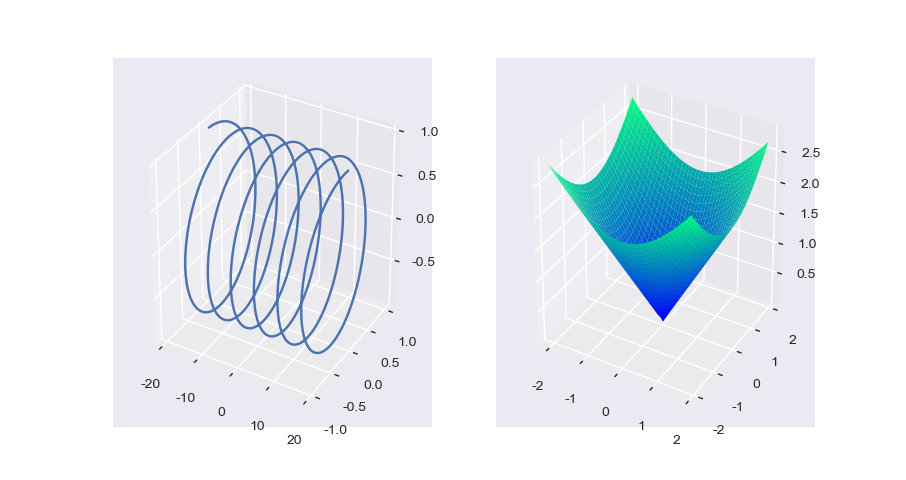• 微信公众号
• 关注微信公众号
•• QQ群
• 我们的QQ群号
•• 本文由 发表于 2018年4月30日20:03:22
• 转载请务必保留本文链接：https://mathpretty.com/9311.html